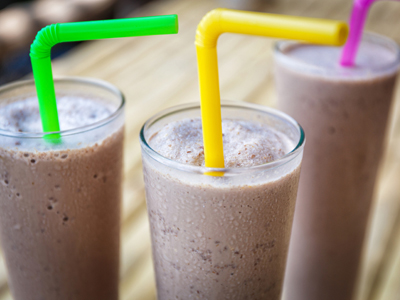King Henry Doesn't Usually Drink Chocolate Milk!

# Measurement - The Metric System - An Introduction

This Math quiz is called 'Measurement - The Metric System - An Introduction' and it has been written by teachers to help you if you are studying the subject at middle school. Playing educational quizzes is a fabulous way to learn if you are in the 6th, 7th or 8th grade - aged 11 to 14.

It costs only \$12.50 per month to play this quiz and over 3,500 others that help you with your school work. You can subscribe on the page at Join Us

Just when you feel like you have mastered math, it seems that teachers throw you another curve ball. It helps keep you on your toes and helps to prepare you for life and the world ahead. So here we are once again delving into another area of math known as the metric system.

If you were born in a country other than the U.S., Liberia or Burma, you would have grown up using the metric system. In reality, it is an easier system to work with than the U.S. system, also known as the American system or the English system. It is easier because it works in base 10 conversions.

The metric system is a set of standardized units of measurement based on the number 10. Why 10? Well, it is because humans have 10 fingers. It is also the system that is virtually used by every scientist in the world, as well as the medical profession. The metric system uses “meters” to measure length and distance, “grams” to measure mass or weight, and “liters” to measure volume.

See below for an example before you tackle the quiz!

Go straight to Quiz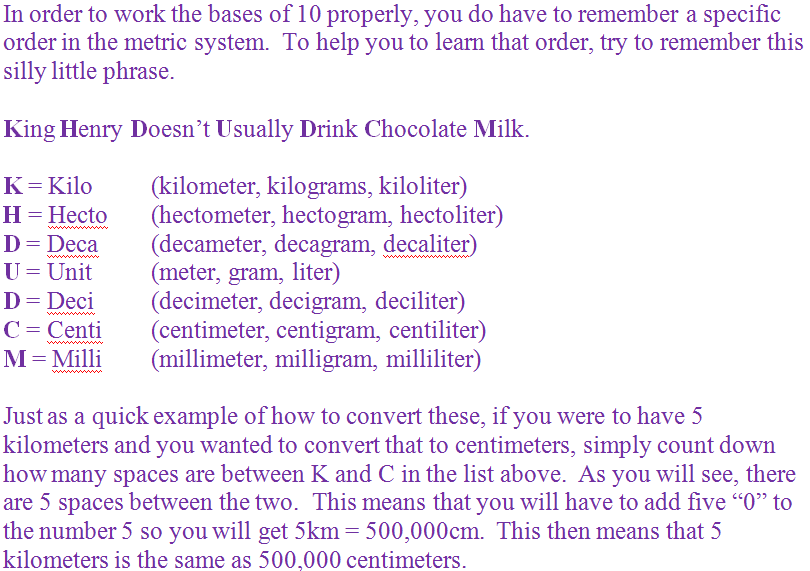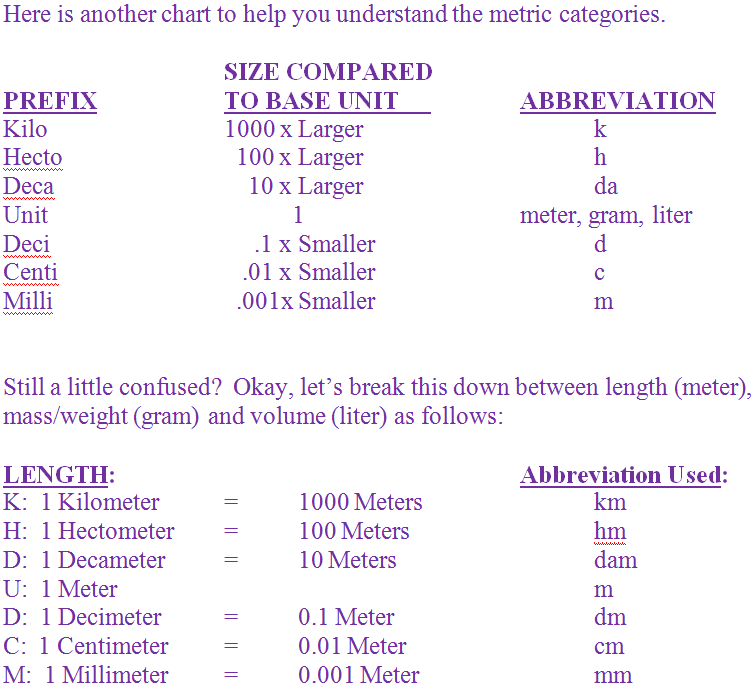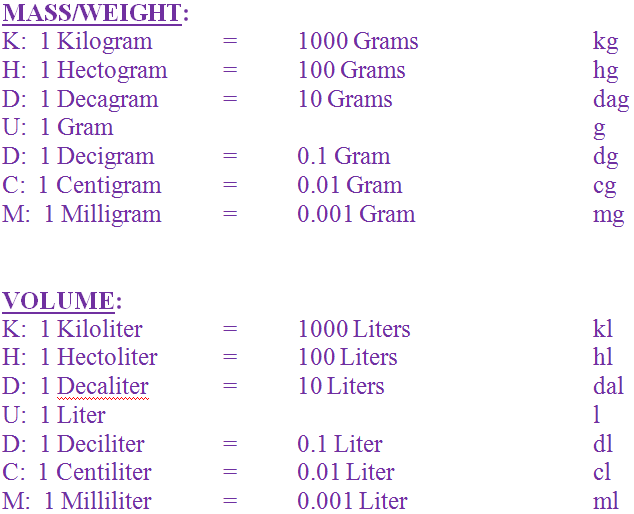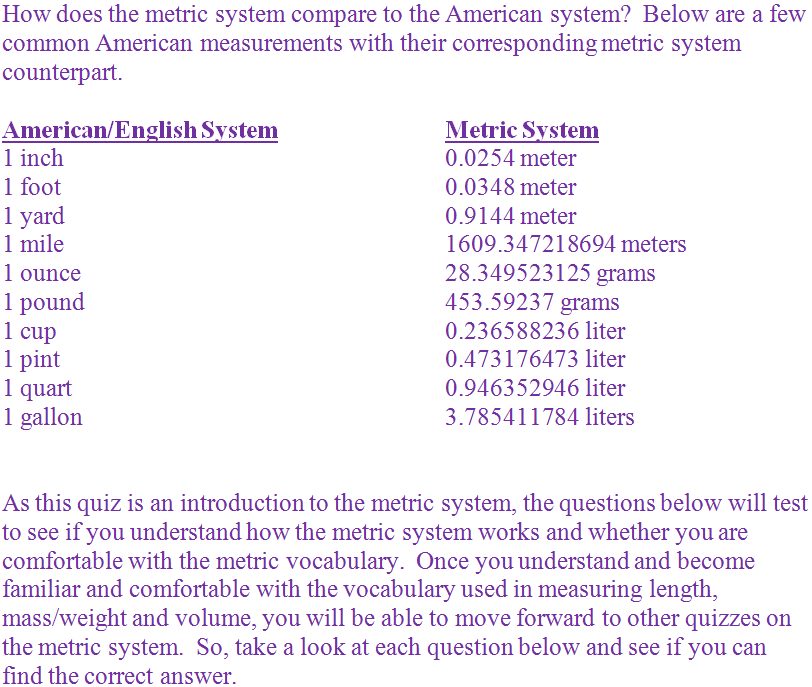1.
The volume of liquids are measured in _____ in the metric system?
grams
Celsius
meters
liters
In the metric system, volume, such as liquids, are measured in liters. Answer (d) is the correct answer
2.
Which word below is NOT a part of the metric system?
decaliter
milligram
centimeter
Although the word quadrameter sounds like it could be related to the metric system, it is actually to do with poetry!
3.
This is a set of standardized units of measurement based on the number 10.
American system
Universal system
Metric system
English system
The metric system is a set of standardized units of measurement based on the number 10. Answer (c) is the correct answer
4.
1 pound is equal to _______ grams.
453.59237
353.59237
253.59237
153.59237
1 pound is equal to 453.59237 grams. Answer (a) is the correct answer
5.
The length of a football field is measured in _____ in the metric system?
liters
yards
meters
grams
In the metric system, length and distances are measured in meters. Answer (c) is the correct answer
6.
Which order of letters below is the correct order of the metric system’s category of measurements?
KHCDUMD
KHDUDCM
KDUCMDH
KCUDHMD
Remember the silly phrase of “King Henry Doesn’t Usually Drink Chocolate Milk.” This tells us the order is KHDUDCM so Answer (b) is the correct answer
7.
1 mile equals _______ meters.
1609.647218694
1609.547218694
1609.447218694
1609.347218694
8.
Which profession below does NOT use the metric system?
Doctors
Lawyers
Scientists
Astronauts
Doctors, scientists and astronauts all use the metric system. Only lawyers do not use the system. Answer (b) is the correct answer
9.
The weight of a can is measured in _____ in the metric system?
grams
liters
bundles
meters
When measuring the weight or mass of an object in the metric system it is measured in grams. Answer (a) is the correct answer
10.
1 gallon equals _______ liters.
1.785411784
2.785411784
3.785411784
4.785411784